## Hundreds Chart Missing Numbers

### Reversed Hundreds Chart With Missing Numbers

What I also wanted was to be able to have a reversed hundreds chart. I was trying to figure out a way to create using Excel but although I could create the numbers, I couldn’t figure out how how to get the missing numbers.

Then I found this site.  It gives lots of different options.

You can have straightforward missing numbers and there are lots of fonts and colours to choose from. Then you can just click randomise to give you lots of different grids using the same options.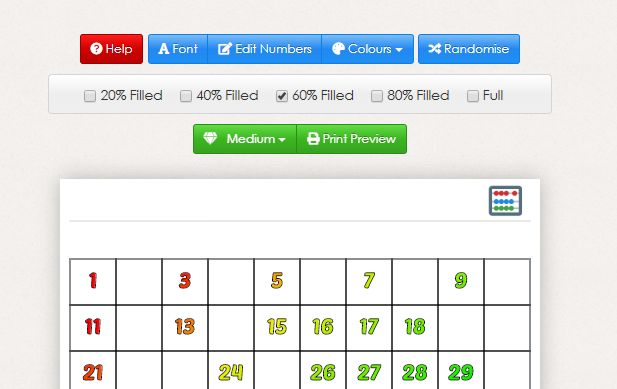However, it is the “Edit Numbers” bit that really excites me!

Click on that and you get this pop up: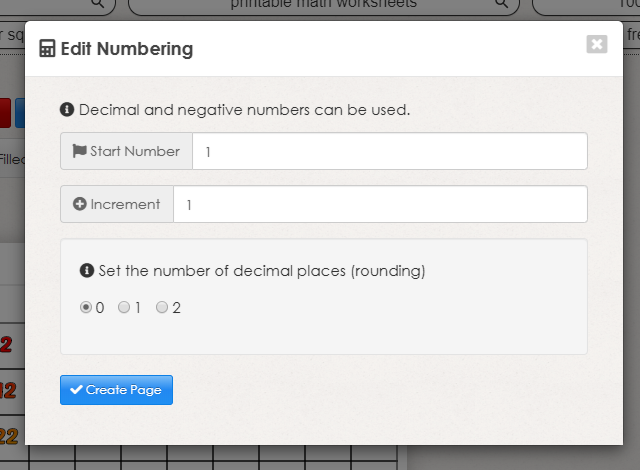As you can see, you can use negative numbers and decimals and even change the increments. So by starting at 100 and setting the increments at -1, I have what I am after.

https://www.senteacher.org/printables/Mathematics/37/HundredSquarePrinter.html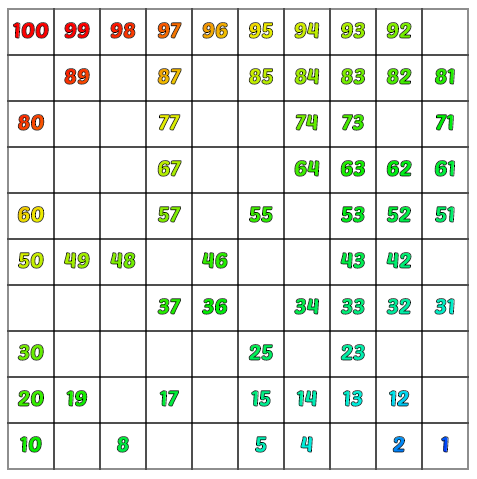### Multiplication tables

4x Table

By starting at 4 and choosing an increment of 4, I can take the 4 x table all the way to 100 x 4.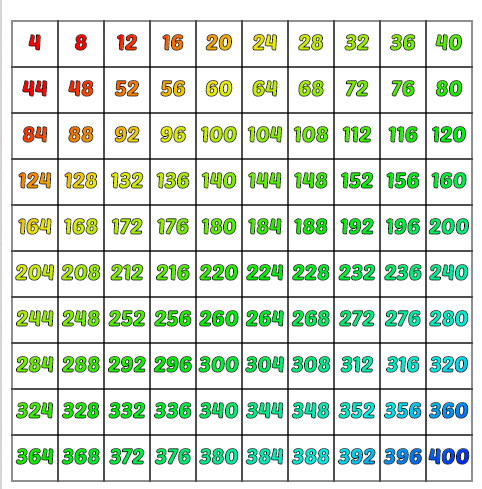One thing that struck me by looking at it, in this format, was that I realised why the 4 x table has the last digit 4,8,2,6,0, pattern running through it.  4×5=20 and so you are adding 20 to 2 to get to 24, 20 to 8 to get to 28, 20 to 12 to get to 32 and so on.

What else might your children notice?

In the table are the numbers: 12, 112, 212, 312.  Would 412 be there if we carried on? Why or why not?

11 x table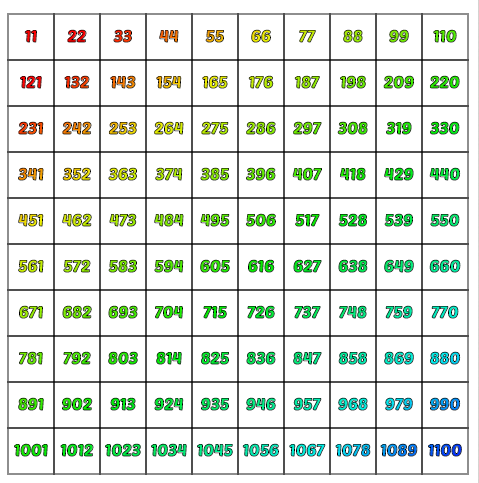What do you notice here?

Take a look at any 3-digit answer where the two outside numbers add up to the number in the centre.  In all these cases the two outside numbers will be the number of times 11 goes into the three-digit number.

For example,  594, 5+4=9,   and 54×11=594

If you have a 3-digit number where the two outside numbers do not add up to the number in the centre, then take away 1 from the first digit in order to work out how many times 11 goes into the whole number.

For example, 836,   8+6=14, so take 1 away from the first digit,  76×11=836.

You can use this information to help you you multiply two-digit numbers by 11.

For example 35,   add 3 and 5 together to make 8 and your answer will be 358.  so 35 x 11 equals 358.

For example 38,  add 3 and 8 together to make 11 and your answer will be 418.   What you did here was to put the 11 in between these two numbers, but then to add Decrease the number in the hundreds column by 1. After all, multiplying by 11 is just multiplying by 10 and then multiplying by 1.By doing this as a column addition and you’ll see what I mean.

380 +

38

Take a look at some of the other tables and see what else you might notice.  Let me know what you spot in the comments area below.

### Some other websites which allow you to print off a free hundreds chart.

HomeSchoolMath.net

https://www.homeschoolmath.net/worksheets/number-charts.php

On this page, you can make a variety of pre-prepared charts but towards the bottom of the page there is also a “Number Chart Worksheet Generator”.

This allows you to create hundreds charts which skip numbers and you can also choose to highlight every, for example, 5th square.  In this example, I have chosen to start at 3, make my increments 3, and hight every 2nd square.

The obvious thing it shows is the 6 x table, but what else can you see?

I found myself adding up the digits of the answers.  What do you think I discovered?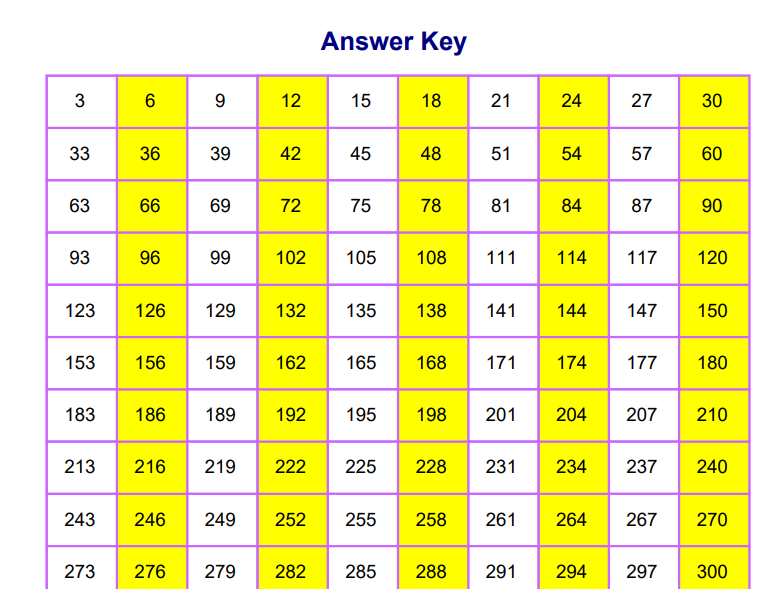### Other types of hundreds charts

One website I enjoy using is http://www.math-aids.com/ . you can use it for free which I did for many years but then decided that there is so much on here to explore that it was really worth paying the subscription fee. This gets rid of all the adverts and gives the site a much cleaner feel.  I think the downloads are quicker as well.

Here are some of the other hundreds charts you can get.

Make puzzles for your kids to complete

http://members.math-aids.com/Hundreds_Chart/Puzzles.html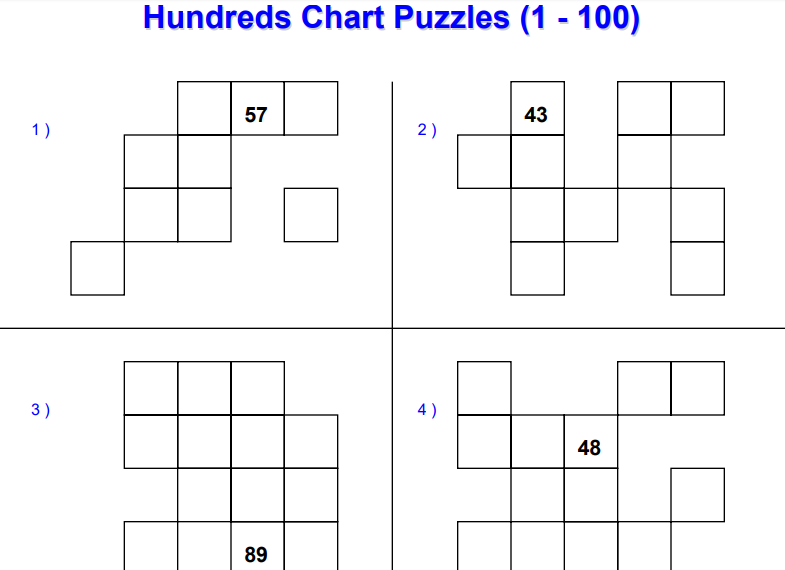### Create pictures by colouring in certain numbers

http://members.math-aids.com/Hundreds_Chart/Pictures.html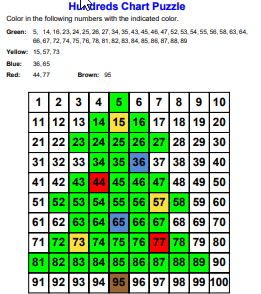### Create a hundreds chart showing whether the round up or down.

http://members.math-aids.com/Hundreds_Chart/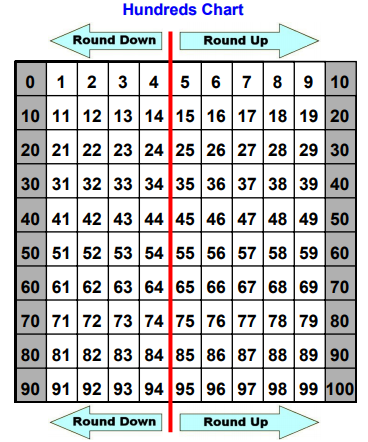### Create letters of the alphabet.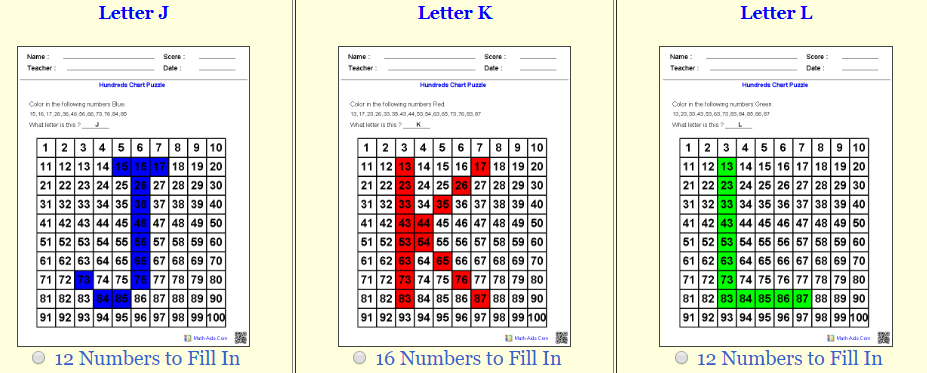Is this useful?  Let me know any ideas of how you might use it.

The one thought I had would be to get children to add up the coloured numbers and see which were the most or the least.

### Some games to play using the hundreds chart

Have a look at this site for some ideas of games to play with hundreds charts.

https://www.thoughtco.com/hundreds-chart-2312157

I would love to find other sites with more ideas on.

Please let me know if you find any.

### Finally, you might want to look at these Youtube videos featuring hundreds charts

Here is a basic introduction to the hundreds chart.

Some basic patterns from Khan Academy

Adding and subtracting by 1 and 10 and how and why to use puzzle parts of the hundreds charts.

Some nice graphics on this video

Number game using puzzle pieces.

Subtraction on the 100s chart

Looking at multiples in the hundreds chart.